In :
from sklearn.datasets import load_breast_cancer
bc = load_breast_cancer()

In :
import pandas as pd
bc_df = pd.DataFrame(bc.data, columns=bc.feature_names)
bc_df.head()

Out:
mean radius mean texture mean perimeter mean area mean smoothness mean compactness mean concavity mean concave points mean symmetry mean fractal dimension ... worst radius worst texture worst perimeter worst area worst smoothness worst compactness worst concavity worst concave points worst symmetry worst fractal dimension
0 17.99 10.38 122.80 1001.0 0.11840 0.27760 0.3001 0.14710 0.2419 0.07871 ... 25.38 17.33 184.60 2019.0 0.1622 0.6656 0.7119 0.2654 0.4601 0.11890
1 20.57 17.77 132.90 1326.0 0.08474 0.07864 0.0869 0.07017 0.1812 0.05667 ... 24.99 23.41 158.80 1956.0 0.1238 0.1866 0.2416 0.1860 0.2750 0.08902
2 19.69 21.25 130.00 1203.0 0.10960 0.15990 0.1974 0.12790 0.2069 0.05999 ... 23.57 25.53 152.50 1709.0 0.1444 0.4245 0.4504 0.2430 0.3613 0.08758
3 11.42 20.38 77.58 386.1 0.14250 0.28390 0.2414 0.10520 0.2597 0.09744 ... 14.91 26.50 98.87 567.7 0.2098 0.8663 0.6869 0.2575 0.6638 0.17300
4 20.29 14.34 135.10 1297.0 0.10030 0.13280 0.1980 0.10430 0.1809 0.05883 ... 22.54 16.67 152.20 1575.0 0.1374 0.2050 0.4000 0.1625 0.2364 0.07678

5 rows × 30 columns

In :
from sklearn.model_selection import train_test_split
X_train, X_test, Y_train, Y_test = train_test_split(bc_df, bc.target, test_size = 0.2, random_state = 31)
print(X_train.shape)
print(Y_train.shape)
print(X_test.shape)
print(Y_test.shape)

(455, 30)
(455,)
(114, 30)
(114,)

In :
from aix360.algorithms.rbm import FeatureBinarizer
fb = FeatureBinarizer(negations=True)
X_train_fb = fb.fit_transform(X_train)
X_test_fb = fb.transform(X_test)
X_train_fb['mean radius'][:8]

Out:
operation <= >
value 10.254 11.328 11.942 12.604 13.270 14.142 15.058 17.026 19.324 10.254 11.328 11.942 12.604 13.270 14.142 15.058 17.026 19.324
468 0 0 0 0 0 0 0 0 1 1 1 1 1 1 1 1 1 0
179 0 0 0 0 1 1 1 1 1 1 1 1 1 0 0 0 0 0
114 1 1 1 1 1 1 1 1 1 0 0 0 0 0 0 0 0 0
35 0 0 0 0 0 0 0 1 1 1 1 1 1 1 1 1 0 0
88 0 0 0 1 1 1 1 1 1 1 1 1 0 0 0 0 0 0
507 0 1 1 1 1 1 1 1 1 1 0 0 0 0 0 0 0 0
213 0 0 0 0 0 0 0 0 1 1 1 1 1 1 1 1 1 0
134 0 0 0 0 0 0 0 0 1 1 1 1 1 1 1 1 1 0
In :
from aix360.algorithms.rbm import GLRMExplainer, LogisticRuleRegression

In :
logistic_model = LogisticRuleRegression(maxSolverIter=2000)
explainer = GLRMExplainer(logistic_model)
explainer.fit(X_train_fb, Y_train)

In :
Y_pred = explainer.predict(X_test_fb)

In :
from sklearn.metrics import accuracy_score, precision_score, recall_score, f1_score
print(f'Accuracy = {accuracy_score(Y_test, Y_pred)}')
print(f'Precision = {precision_score(Y_test, Y_pred, average="macro")}')
print(f'Recall = {recall_score(Y_test, Y_pred, average="macro")}')
print(f'F1 = {f1_score(Y_test, Y_pred, average="macro")}')

Accuracy = 0.9122807017543859
Precision = 0.9271794871794872
Recall = 0.8967926325817719
F1 = 0.9066491975106452

In :
explainer.explain()

Out:
rule coefficient
0 (intercept) -11.1496
1 worst perimeter <= 116.46 AND worst concave po... -11.9584
2 worst concave points <= 0.15 10.1245
3 worst perimeter <= 116.46 AND worst concave po... 9.8396
4 worst area <= 930.88 5.40176
5 worst area > 680.60 AND worst concavity > 0.22 -3.33913
6 worst perimeter <= 116.46 AND worst smoothness... 3.13943
7 mean concave points <= 0.05 1.51205
8 worst concavity <= 0.27 0.925846
9 worst concave points <= 0.12 0.633953
10 worst perimeter <= 104.38 -0.0207581
In :
%matplotlib inline

fb = FeatureBinarizer(negations=True)
figs, _ = explainer.visualize(bc_df, fb)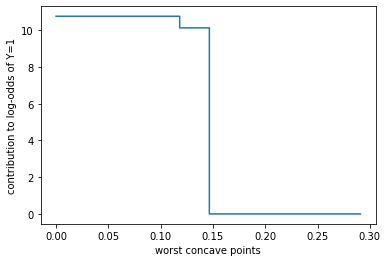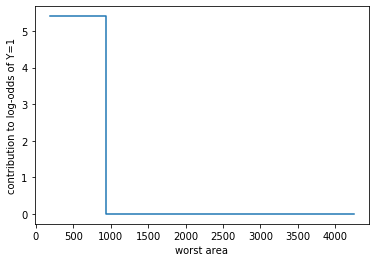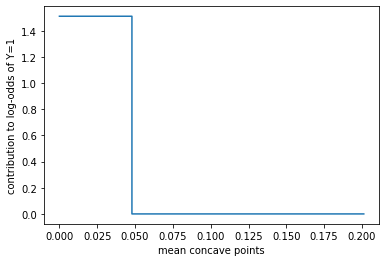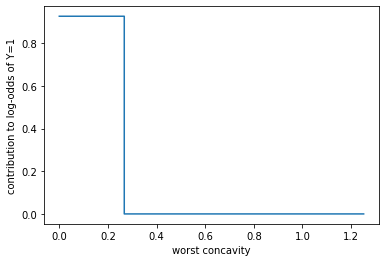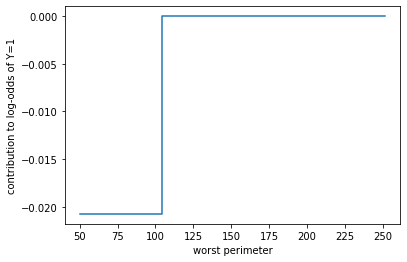In [ ]: